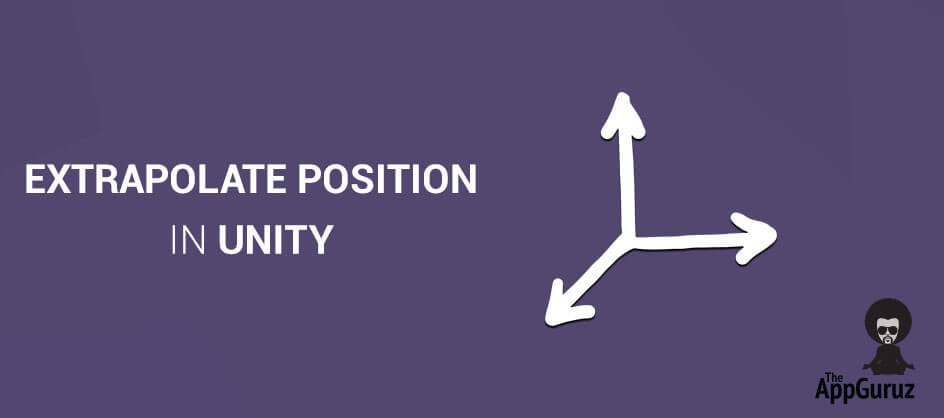## Objective

Main objective of this blog post to give you an idea about how to extrapolate position in unity.

Newton's first law of motion:

“An object at rest stays at rest and an object in motion stays in motion with the same speed and in the same direction unless acted upon by an unbalanced force.”

## Step 1 Newton's First Law

Test Newton's first law of motion In Unity:

1. Take two cubes and name them as CubeUnderUnityPhysics and ExtrapolatedCube.
2. Add rigid body component to CubeUnderUnityPhysics.
3. Create an empty game object named PhysicsTest and add script PhysicsTest to it and set both, cube reference and initial velocity of it.

No unbalanced force:

The only condition for this law is no unbalanced force should act upon it. Therefore set gravity and drag it to zero. Gravity can be changed from Edit->Project Settings->Physics.

Here, CubeUnderUnityPhysics acts under unity physics engine and position of ExtrapolatedCube is updated by the script and gives its position at any time after the initial velocity is given.

We know that,

Velocity = distance / time

``````dx=v0x* t
dy=v0y* t
dz=v0z* t``````

Under this circumstance the position of ExtrapolatedCube is defined by,

``````ExtrapolatedCube.position.x = initialVeiocity.x * Time.time;
ExtrapolatedCube.position.y = initialVeiocity.y * Time.time;
ExtrapolatedCube.position.z = initialVeiocity.z* Time.time;``````

Therefore, CubeUnderUnityPhysics and hence ExtrapolatedCube has constant velocity and no acceleration.

## Step 2 Apply Gravity

But what if gravity is applied?

For constant acceleration motion,

``d= vo t + a t2/2``

We know that the gravity of earth is -9.8 m/s2.It means that at every second the velocity is increased by 9.8 in the downward direction.

Gravity is in the downward direction. So, only the y component changes.

``````dx=v0x * t
dy=v0y * t - 9.81 * t2 / 2
dz=v0z * t``````

For position of ExtrapolatedCube,

``````ExtrapolatedCube.position.x = initialVeiocity.x * Time.time;
ExtrapolatedCube.position.y = initialVeiocity.y * Time.time +9.81f * Time.time * Time.time / 2 ;
ExtrapolatedCube.position.z = initialVeiocity.z* Time.time;``````

Note: Make sure that the gravity on Edit->Project Settings->Physics is (0,-9.81,0).

## Step 3 Change in Gravity

What if the gravity is other than (0,-9.81, 0)?

Suppose we have a game in which the gravity is other than (0,-9.81, 0).

We need to rewrite the above equation as given below,

``````ExtrapolatedCube.position.x = initialVeiocity.x * Time.time+Physics.gravity.x* Time.time * Time.time / 2;
ExtrapolatedCube.position.y = initialVeiocity.y * Time.time +Physics.gravity.y * Time.time*Time.time / 2;
ExtrapolatedCube.position.z = initialVeiocity.z* Time.time+Physics.gravity.z* Time.time * Time.time / 2;``````

## Step 4 Initial Position is different

What if the initial position of the object is not (0, 0, 0)?

In this case the equation should be,

``````ExtrapolatedCube.position.x =initialPosition.x+ initialVeiocity.x * Time.time;
ExtrapolatedCube.position.y=initialPosition.y+initialVeiocity.y*Time.time +9.81f * Time.time * Time.time / 2;
ExtrapolatedCube.position.z =initialPosition.z + initialVeiocity.z* Time.time;``````

## Step 5 Extrapolate position after the particular time

Until now we’ve used time.time as time because we were interested in comparing both the cube positions.

Now, if we want to know the position of CubeUnderUnityPhysics after any particular time for example after 2 s, then use the following equations:

``````ExtrapolatedCube.position.x = initialPosition.x+ currentVeiocity.x * 2;
ExtrapolatedCube.position.y=initialPosition.y+ currentVeiocity.y*2 + 9.81f * 2 * 2 / 2;
ExtrapolatedCube.position.z = initialPosition.z + currentVeiocity.z* 2;``````

I hope you find this blog post very helpful while working on Extrapolate Position in Unity. Let me know in comment if you have any questions regarding unity. I will reply you ASAP.

Got an idea of unity game development what are you still waiting for? Contact us now and see the idea live soon. Our company has been named as one of the best game development company in India.I am Professional Game Developer, I love to develop a Game and also i love play a game, And i am always ready to helping you in Game Development.